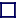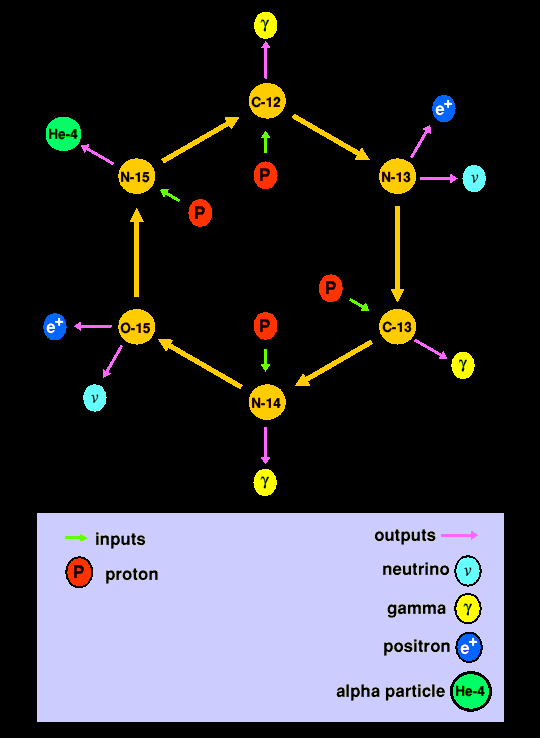### CNO CYCLE

The CNO cycle is the carbon, nitrogen, oxygen cycle of nucleosynthesis, for which the reaction rate is hypercritically dependant upon the temperature, being proportional to the 17th power of the temperature. The CNO cycle within the sun does take place, but because the suns' core is only at 15.57 million Kelvin, the reaction is slow. If the sun were twice as massive, the CNO cycle would predominate. In essence the CNO cycle uses carbon to catalyse the formation of helium-4. It proceeds through the successive addition of protons to carbon-12, with two intermediate inverse beta decay events effecting the conversion of two of the four input protons into neutrons.

 1) C-12 + p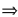N-13 +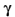2) N-13C-13 + e+ +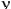(inverse beta decay) 3) C-13 + pN-14 +4) N-14 + pO-15 +5) O-15N-15 + e+ +(inverse beta decay) 6) N-15 + pHe-4 + C-12

These reactions increase the abundance of C-12, C-13, N-14, N-15 and O-15 in stars.